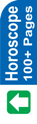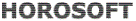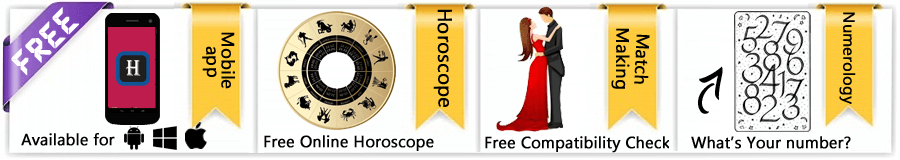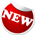Astrology Software
Indian AstrologyCalculation of Ascendant For making the horoscope, first of all we have to calculate the Ascendant. Ascendant is the rising sign at the time of birth. Let us see how the Ascendant is calculated. This will be done with the help of two books, viz. Table of Ascendants, and Annual Ephemeries, 1993 (for the year of birth) by Lahiri. These two books can be procured from the market. Details of sample birth are once again given below. We would calculate Ascendant for this. Date of birth : 10.12.1993, Time of birth : 3.50 AM IST, and Place of birth : Bombay. We have indicated the Longitude and Latitude of Bombay as above. These were taken from page 101 of the book "Table of Ascendants". This book contains the necessary information about many major cities of India and abroad. If the city required is not given therein, kindly see the Atlas for these details. And, if Atlas is not handy, nearest city can be taken. But for accurate casting of horoscope, accurate details are required. As made clear earlier, in a northern horoscope, Ascending sign is written in the top centre and then zodiac signs are continued anti-clockwise. Later on planets are placed in the horoscope. So, to calculate the Ascendant sign, the first Step is to find out the Local Mean Time (LMT). Step 1 Method 1 Convert the IST to LMT. There are two ways to find out the correction time to IST to obtain LMT:: i. Take the Longitude of Bombay, i.e. 72° 50' N. Formula for getting the correction time to IST to find out LMT is 15° = 1 hour, or 1° = 4 minutes (Please note, Longitude is given in degrees (°), minutes (') and seconds (''), [ 1° = 60', 1' = 60''), whereas the time is given in hrs, minutes and seconds [1 hr = 60 minutes, 1 minute = 60 seconds]. Do not confuse the minutes of Longitude with the minutes of time. As per above formula, divide Longitude 72° 50' by 15 to know the LMT. It comes to: 4 hr 51 mt 20 secs. How? This is shown below to facilitate your understanding of the calculations. {72°/15 = 4 12/15 (i.e. 4 hrs + 12/15 hrs) 12/15 x 60 (to make minutes) = 48 minutes Hence, 4 hr 48 minutes Now 50' /15 = 3 5/15 (i.e. 3 minutes + 5/15 or 1/3 minutes). 1/3 x 60 (to make seconds) = 20 seconds. Hence, 4 hrs, 48+3 minutes, 20 seconds. = 4 h 51 m 20 s. Now deduct 5 h 30 m 0 s (as GMT is +5h 30 m 0 s of IST) from 4 h 51m 20s: 4 h 51 m 20 s - 5 h 30 m 0 s - 0 h 38 m 40s This means, 38 m 40 s should be deducted from IST to find LMT. Method 2 Formula: 4 x (Longitude of the city - 82° 30') = 4 (72° 50'- 82° 30') = 4 ( - 9° 40') Hence 4 x 9 = 36 m and 4 x 40 = 160" = 160/60' = 2' 40" Therefore, 36 m + 2 m 40 s = 38 m 40 s Since this answer is in negative, deduct 38 m 40 s from IST to obtain LMT. Method 3 Refer to Table of Ascendants, page 101. Against Bombay, Local Time correction to IST is given as -38 m 40 s (which is same as calculated by us). Use of Book is easy and quick. The first and second methods have been given only for understanding. Hence, LMT for the required time of birth is: IST: 3 h 50 m 0 s Time correction: -0 h 38 m 40 s 3 h 11 m 20s ............A Step II The next step is to find out the sidereal time for the given date, time and place of birth. Sidereal time for 10th December (From page 3, Table of Asc) 17 h 14 m 07 s Correction to Indian sidereal time for Bombay (From page 101, last column, Table of Asc) + 06 s Correction for 1993 (From page 4, Table II, Table of Asc) + 1 m 52 s Sidereal time for 12 Noon on 10.12.1993 for Bombay 17 h 16 m 05s ..B Now, let us calculate sidereal time for the time of birth on 10.12.1993 for Bombay: As the birth is before 12 Noon, deduct from 12 h 00 m 00 s LMT 3 h 11 m 20 s 8 h 48 m 40 s ... C (For afternoon birth, there is no need of this deduction. Correction to increase the time interval is apllied straight to the LMT). Now apply correction to increase the time interval (From page 5, Table IV, Table of Asc): for 8 hr + 1 m 19 s for 48 m + 8 s for 8 hr 48 m: 1 m 27 s ..... D Now, add C and D. This comes to 8 h 50 m 07 s. Sidereal time at 12 Noon 17 h 16 m 05 s Less corrected time interval - 8 h 50 m 07 s (For birth in the afternoon, this should be added.) Sidereal Time for the given 8 h 25 m 58 s ...E birth time: Step III Now to find out the Ascendant, refer to Tables given in the book Table of Ascendants for various latitudes. Search for the latitude of Bombay (or its nearest place, if the same is not available). On page 29, Table for Latitude 19° N is given. As given earlier, the latitude for Bombay, the required place of birth, is 18° 58' N (say, 19° N). In this page, we have to search for the Ascendant for the Sidereal Time given above as, 8 hr 25 m 58 s as at E. Ascendant for For 8 h 24 m 6 s 100 46' For 8 h 28 m 6s 110 41' Hence for 4 m 55' ('s' is the zodiac sign, Degree (°) and minute (') already explained above). If we deduct the former from the latter, we get 4 minutes = 55 m but we need 2 minutes (8h 24 m + 1 m 58' (1 m 58' is approximately equal to 2') = 8 h 26 m. So for 2 minutes, we get 28 m (55/2: 27.5, say 28). Therefore, Ascendant for 8 h 25 m 58 s (Or say, 8 h 26 m) = 6 s 10° 46' + 28' 6 s 110 14' Step IV Now, apply correction for Ayanamasha for 1993, as per page 6 of Tables of Ascedants, which is -46'. Therefore, Ascendant for the required birth: 6 s 11° 14' - 46' Ascendant(I): 6s 10° 28' This means, Ascending sign is Libra (7), and it has travelled 10° 28'in Libra. Horoscope for this birth will be caste by writing 7 in the top centre, as explained earlier.Astrowealth Astrology Software Software for Website Astrology Reports Free Services Regional Language Important Link